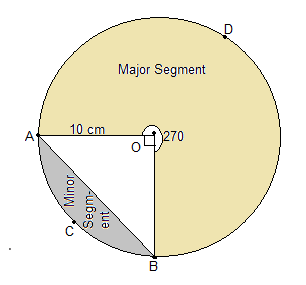# A chord of a circle of radius 10 cm subtends a right angle at the centre. Find the area of the corresponding: (i) minor segment (ii) major sector. (Use π = 3.14)

Given

A chord of a circle of radius 10 cm subtends a right angle at the centre.

Find out

We have to find out the area of the corresponding:

(i) minor segment

(ii) major sector. (Use π = 3.14)

SolutionThe major segment is =360°-90°=270°

(i) Area of minor segment =Area of sector – Area of the triangle

=∅/(360°) *πr2-1/2*h*b

=90/306*3.14*10*10-1/2*10*10

=314/4-50

=78.5-50

= 28.5 cm2

(ii)Area of major sector=∅/(360°) *πr2

=270/360*3.14*10*10

=3*314/4=235.5cm2

Area of major segment=235.5cm2(9)(0)